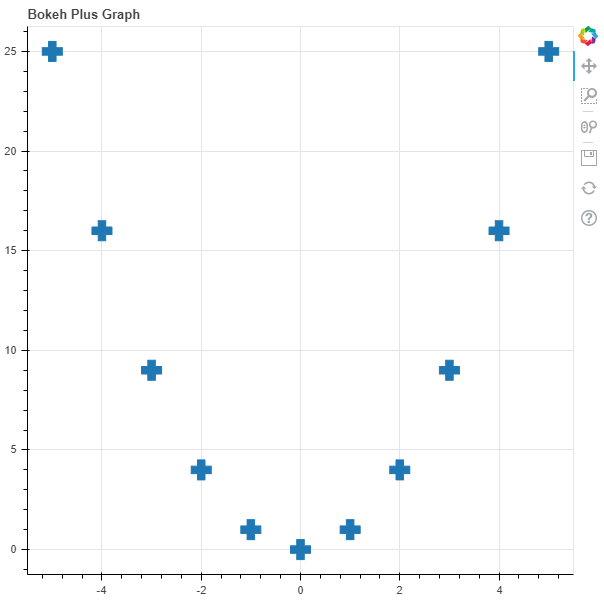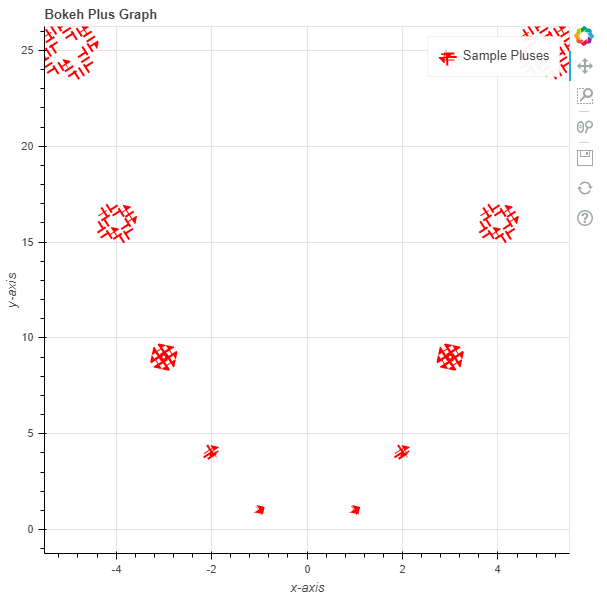Related Articles

# Python Bokeh – Plotting Pluses on a Graph

• Last Updated : 10 Jul, 2020

Bokeh is a Python interactive data visualization. It renders its plots using HTML and JavaScript. It targets modern web browsers for presentation providing elegant, concise construction of novel graphics with high-performance interactivity.

Bokeh can be used to plot pluses on a graph. Plotting pluses on a graph can be done using the `plus()` method of the `plotting` module.

## plotting.figure.plus()

Syntax : plus(parameters)

Parameters :

• x : x-axis coordinates of the center the plus markers
• y : y-axis coordinates of the plus markers

Returns : an object of class `GlyphRenderer`

Example 1 : In this example we will be using the default values for plotting the graph.

 `# importing the modules``from` `bokeh.plotting ``import` `figure, output_file, show``      ` `# file to save the model``output_file(``"gfg.html"``)``      ` `# instantiating the figure object``graph ``=` `figure(title ``=` `"Bokeh Plus Graph"``)``    ` `# the points to be plotted``x ``=` `[``-``5``, ``-``4``, ``-``3``, ``-``2``, ``-``1``, ``0``, ``1``, ``2``, ``3``, ``4``, ``5``]``y ``=` `[i ``*``*` `2` `for` `i ``in` `x]``   ` `# plotting the graph``graph.plus(x, y, size ``=` `20``)``    ` `# displaying the model``show(graph)`

Output :Example 2 : In this example we will be plotting the pluses with dotted lines alongside other parameters and the size of the hexagons are in proportion to their values.

 `# importing the modules``from` `bokeh.plotting ``import` `figure, output_file, show``     ` `# file to save the model``output_file(``"gfg.html"``)``     ` `# instantiating the figure object``graph ``=` `figure(title ``=` `"Bokeh Plus Graph"``)``   ` `# name of the x-axis``graph.xaxis.axis_label ``=` `"x-axis"``    ` `# name of the y-axis``graph.yaxis.axis_label ``=` `"y-axis"``   ` `# the points to be plotted``x ``=` `[``-``5``, ``-``4``, ``-``3``, ``-``2``, ``-``1``, ``0``, ``1``, ``2``, ``3``, ``4``, ``5``]``y ``=` `[i ``*``*` `2` `for` `i ``in` `x]``   ` `# size of the hexagons``size ``=` `[i ``*` `2` `for` `i ``in` `y]``   ` `# angle of the hexagons``angle ``=` `10``  ` `# fill color value``fill_color ``=` `None``  ` `# color of the line``line_color ``=` `"red"``    ` `# type of line``line_dash ``=` `"dotted"``    ` `# offset of line dash``line_dash_offset ``=` `1``   ` `# width of the dashes``line_width ``=` `10``   ` `# name of the legend``legend_label ``=` `"Sample Pluses"``    ` `# plotting the graph``graph.plus(x, y,``           ``size ``=` `size,``           ``angle ``=` `angle,``           ``fill_color ``=` `fill_color,``           ``line_color ``=` `line_color,``           ``line_dash ``=` `line_dash,``           ``line_dash_offset ``=` `line_dash_offset,``           ``line_width ``=` `line_width,``           ``legend_label ``=` `legend_label)``   ` `     ` `# displaying the model``show(graph)`

Output :Attention geek! Strengthen your foundations with the Python Programming Foundation Course and learn the basics.

To begin with, your interview preparations Enhance your Data Structures concepts with the Python DS Course. And to begin with your Machine Learning Journey, join the Machine Learning – Basic Level Course

My Personal Notes arrow_drop_up